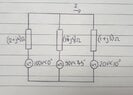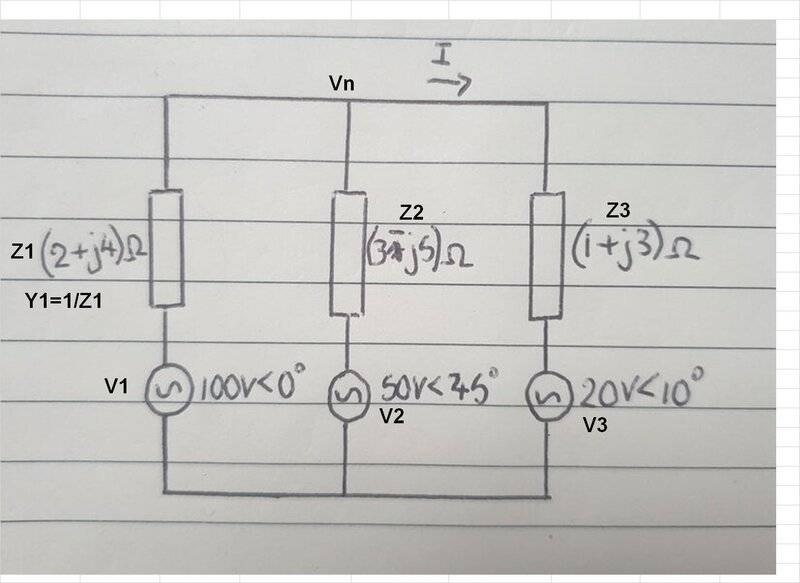# Use voltage nodal analysis to solve for the current flowing in this AC circuit

• Engineering
• fantis3

#### fantis3

Homework Statement
Use a voltage nodal analysis to solve the current I flowing in the AC circuit
below. Draw the circuit with assumed nodes and write down the nodal
equations. Draw the phasor diagram and show the position of the current in the
diagram with reference to the voltage 100V
Relevant Equations
I=V/R
Hi first time using this forum, I have some homework that I am entirely stuck on, can anyone help me with this question? I understand the placement of nodes, and that I need to convert complex numbers, but apart from that I have no idea what direction that I'm supposed to take in this. I don't expect an answer to any of the questions, I'd just appreciate a push in the right direction if anyone can help. I've also attached a drawing of the circuit diagram below

#### Attachments

•20221116_160242.jpg
33.9 KB · Views: 46
You need to identify all the nodes, choose the ground node (0V point) and write the voltages of the nodes w.r.t the ground node.

Have you done any nodal analysis problems for dc circuits before?

At first you have to find the potential Vn of the star neutral using formula Vn=(V1*Y1+V2*Y2+V3*Y3)/(Y1+Y2+Y3) and then I1=(V1-VN)/Z1 and so on.